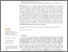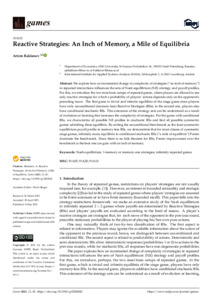Reactive Strategies: An Inch of Memory, a Mile of Equilibria

(2021). Reactive Strategies: An Inch of Memory, a Mile of Equilibria. Games 12 (2) e42. 10.3390/g12020042.Preview
Text
games-12-00042-v3.pdf - Published Version

Abstract

We explore how an incremental change in complexity of strategies (“an inch of memory”) in repeated interactions influences the sets of Nash equilibrium (NE) strategy and payoff profiles. For this, we introduce the two most basic setups of repeated games, where players are allowed to use only reactive strategies for which a probability of players’ actions depends only on the opponent’s preceding move. The first game is trivial and inherits equilibria of the stage game since players have only unconditional (memory-less) Reactive Strategies (RSs); in the second one, players also have conditional stochastic RSs. This extension of the strategy sets can be understood as a result of evolution or learning that increases the complexity of strategies. For the game with conditional RSs, we characterize all possible NE profiles in stochastic RSs and find all possible symmetric games admitting these equilibria. By setting the unconditional benchmark as the least symmetric equilibrium payoff profile in memory-less RSs, we demonstrate that for most classes of symmetric stage games, infinitely many equilibria in conditional stochastic RSs (“a mile of equilibria”) Pareto dominate the benchmark. Since there is no folk theorem for RSs, Pareto improvement over the benchmark is the best one can gain with an inch of memory.

Item Type: Article 1-memory or memory-one strategies; Infinitely repeated games; Nash equilibrium Advancing Systems Analysis (ASA)Advancing Systems Analysis (ASA) > Exploratory Modeling of Human-natural Systems (EM) Luke Kirwan 07 Jun 2021 07:24 27 Aug 2021 17:34 http://pure.iiasa.ac.at/17242View Item